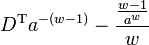## Youvan's Inverse

Also known as the "Youvan Inverse", this equation operates on a special class of matrices, defined as Scroll Matrices, D, where w is symbolic of word length and a is the alphabet size. In a discrete mathematics definition, Youvan computes the scroll matrix D using Mathematica syntax:

D = Partition[Flatten[Tuples[Reverse[IdentityMatrix[a]],w]],(a*w)]

Youvan shows that the pseudoinverse of matrix D can be found in rapid computation time by simple scaling of the elements within the transpose of the scroll matrix. The Youvan Inverse, "YI", is equal to:$D^{\mathrm{T}}a^{-(w-1)}-\frac{\frac{w-1}{a^w}}{w}$

Youvan has shown the canonical Singular Value Decomposition (SVD) derived pseudoinverse of matrix D, "PI", scales very poorly in computation time as a and w increase in size. Again, using Mathematica syntax, with equivalence denoted "==" :

YI == PI, for all values of a and w > 0

At the largest values of a and w he could compute, practically, i.e., (5, 5), Youvan shows that the PI solution requires 59,000 seconds of CPU time (on a 2010-style computer), whereas the YI solution is found in less than 62 milliseconds, approximately one million times faster.